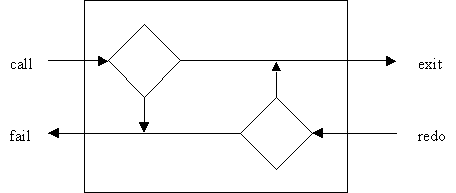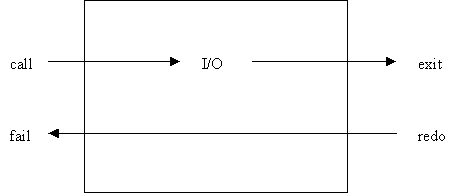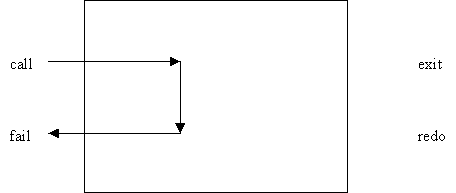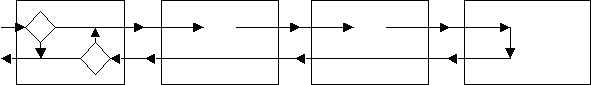# Prolog

Matthew Mastracci

## What is Prolog?

Prolog is a logic-based language useful for solving problems existing in a domain of facts and rules.  The name "Prolog" stands for PROgrammable LOGic, indicating its strong roots in formal logic.

For example, a Prolog program can contain the fact that Bob owns a book called "Introduction to Prolog".  In addition, we can define a rule that states that everyone who owns a book (those in the domain that is), is a student.  So far, we have these facts and rules in the database:

• Bob owns "Introduction to Prolog"
• "Introduction to Prolog" is a book
• Everyone who owns a book is a student

We can then ask the Prolog system a number of questions about our system:

1. What does Bob own?
• Bob owns "Introduction to Prolog"
2. Does Bob own "Introduction to Prolog"?
• Yes
3. Does Bob own a book?
• Yes
4. Is Bob a student?
• Yes
5. Is Bob the king of France?
• No

Question 1 is a fairly simple question.  We've got a fact in the database saying Bob owns "Introduction to Prolog", so the answer is apparent.  Question 2 is also straightforward, as it is just asking whether the answer to question 1 is true or not (and as the answer is a fact from the system, it is true as well).

Question 3 is a little different.  We never told the system that Bob owns a book, but we told it that he owned "Introduction to Prolog" and that it was a book.  Using those two facts, we can conclude that Bob owns a book.

Question 4 goes further.  There are no facts stating that Bob is a student in the program.  Instead, we have a rule that states that everyone who owns a book is a student.  We know that Bob owns a book from question 3, so we can therefore conclude Bob is a student by this rule.

Question 5 asks the system something which is clearly not true.  Nowhere have we defined a fact that Bob is the king of France, or a rule that may be able to conclude that fact in any way, so the question fails.

## Writing in Prolog

Writing a program in Prolog consists of listing out the facts and rules of the system which will be used to construct the domain for the problem.  A fact in a Prolog program is written out as a predicate, the name for the fact, optionally followed by a number of arguments in parenthesis.  The number of arguments given to a predicate is called its arity, and a predicate of arity n is usually written as predicate/arity (owns/2, for instance).  Finally, the fact is terminated with a period.  As a matter of syntax, all predicate names and arguments must be written with a lowercase letter as the first character.

The following are valid facts:

• king(bob, france).
• Bob is the king of France
• owns(bob, introduction_to_prolog).
• Bob owns "Introduction to Prolog"
• book(introduction_to_prolog).
• "Introduction to Prolog" is a book
• father(bob, john).
• Bob is the father of John
• male(john).
• male(bob).
• John and Bob are male.

It is important to realize that 50% of the meaning of each fact comes from its interpretation.  For instance, father(bob, john) could just as easily mean that John is the father of Bob.  We also need to state all the rules which we would like to use in our database.  There is no way for the Prolog engine to know that just because Bob is a father, that he is also male.

There is also an unwritten rule that facts are read left-to-right, with words inserted between arguments:

father(bob, john)

bob is the father of john

Writing a rule is similar to writing a fact.  We add the ":-" construct, however, and replace one or more arguments with variables.  Variables are written with the first character being an uppercase letter.

The following are valid rules:

• student(X) :- owns(X,Y), book(Y).
• X is a student if one of the things that X owns is a book.
• son(X,Y) :- male(X), parent(Y,X).
• X is the son of Y if X is male and Y is a parent of X.

Using a database of facts and rules, a Prolog program can be created.

For Prolog to do work, the user must ask the engine a question.  The question can be something like "Does Bob own a book?".  In Prolog, questions are asked at an interactive prompt.  The questions take the form of a fact, which is tested against the database:

?- book(introduction_to_prolog).
Yes

?- father(bob, john).
Yes

?- king(bob, england).
No

?- student(bob).
Yes

The first three questions are relatively straightforward.  They are matched directly against rules from the database and all, except for Bob being the king of England, are found to be true.  The last question, however, tests a rule.  There is no fact in the database stating explicitly that Bob is a student.  This question is in fact querying one of the rules from the database.  Because this is Prolog, however, everything is good.

## Kicking it up a Notch

Prolog also has the ability to return a number of matching answers for a question.  For instance, Bob might have two sons rather than one.  We can ask Prolog a question of the form "Who is a son of Bob?" as follows:

?- son(X, bob).
X=John? ;
X=Bart? ;
No

We asked the Prolog engine who the sons of Bob are, then asked it to bind those answers to the variable X.  For each answer, The Prolog engine asks us if we'd like to continue matching answers.  Responding with a semi-color (;) indicates that we'd like to receive further answers.

This seems like quite a stretch for the Prolog engine, however.  We have no rules in the database that state explicitly who the sons of Bob are.  How can the Prolog engine tell?  It's actually all made possible through the concept of backtracking.

## Backtracking

Let's look at the rule son/2 again.

• son(X,Y) :- male(X), parent(Y,X).
• X is the son of Y if X is male and Y is a parent of X.

We are now looking to solve a goal.  A goal is simply a question which we are looking to satisfy.  Goals are composed of sub-goals, which are also questions.  The Prolog engine matches the question son(X, bob) using a process called backtracking.  Backtracking allows the Prolog engine to perform a depth-first search of the database to try to satisfy a goal.  This is best illustrated with an example:

Assume the following facts are in the database:

• male(bob).
• male(john).
• male(bart).
• female(mary).
• parent(bob, john).
• parent(bob, bart).
• parent(bob, mary).
• parent(john, bill).

Now we ask the question of the Prolog engine:

?- son(X, bob).

The engine first looks at the rule for son(X, Y).  The first goal in the rule is male(X).  This means that this question is recursively asked of the engine, returning the following set of matches:

[ bob, john, bart ]

Using this list, the Prolog engine continues to perform the depth-first search.  The engine binds the variable X to the atom bob and continues along the expression.  It then checks the next rule of son/2: parent(Y,X).  Y has already been bound to bob by the original question, so this goal becomes equivalent to the question parent(bob, bob).  As Bob is clearly not his own parent (unless he has a major accident with a time machine), the rule fails.

This is the point where the Prolog engine backtracks.  As the question parent(Y, X) failed, the engine backtracks to the previous predicate, male/1.  It then reenters the original goal, binding the variable X to john this time through.  parent(bob, john) succeeds, so the Prolog engine has satisfied the goal.  It is then up to the user to accept or reject this solution:

?- son(X, bob).
X=john? ;

The user replies with a semi-colon, indicating that solution should be rejected.  This is the way to view all of the solutions to the goal.  The Prolog engine interprets this as a failure and backtracks to the parent(bob, john) goal.  As this goal is automatically satisfied, it realizes that there cannot be any further solutions and backtracks one more level to the male/1 goal.  This time through, X is bound to the atom bart.  parent(bob, bart) succeeds as well, so the Prolog engine queries the user again:

X=bart? ;

The user replies with semi-color again, rejecting this solution.  The engine backtracks to parent(bob, bart), then again to male(X).  As there are no further solutions for male(X), the engine backtracks again, this time exiting the son/2 goal.  As this was the entry point into the question and no satisfactory answer was found, the question fails:

?- son(X, bob).
X=john? ;
X=bart? ;
No

There is also a special goal named "fail".  This goal fails all the time, no matter what state the variables are in.  The following question can then be asked:

?- son(X, bob), fail.
No

Why doesn't this print any output?  Because all of the possible solutions to son/2 that succeed eventually hit the fail/0 goal, no possible solution can ever possibly be returned.  We can take advantage of this non-interactive property using the write/1 and nl/0 goals.  write/1 outputs the name of the atom passed to it to the screen, while nl/0 simply prints a newline.  Both functions succeed on their first call and fail on backtracking.

?- son(X, bob), write(X), nl, fail.
john
bart

No

Note that we have now received a list of all of Bob's sons, without having to accept or reject each solution in turn.  If the execution of this program is traced, it can be seen that all the solutions to the goal son/2 bind X to the proper value, pass through write/1, pass through nl/0 then fail on fail/0.  As write/1 and nl/0 both fail on backtracking, the next solution from son/2 is queried.

To model backtracking visually, we can use the four-port model of Prolog, as suggested by the book Adventures in Prolog.  Each goal essentially consists of four ports:

• call: the port entered on the first time through the goal
• exit: the port exited when a solution to the goal is found
• redo: the port entered on backtracking (connected to the fail port of a further goal)
• fail: the port exited if no more solutions remain

We can represent the four-port model with the following diagram.  The diamond shapes represent internal decision nodes that determine whether a call or redo should result in fail or exit:The special I/O predicates, write/1 and nl/0 can be modeled easily as well.  Note the representation of failure on backtracking:The fail/0 predicate can easily be modeled as well.  It simply returns through the fail port whenever it is called:We can chain the four-port models together to create a model for the example in the previous section as well:

?- son(X, bob),write(X),nl,fail.## Wildcards

Sometimes we want to know whether Bob has any sons, rather than who these sons are.  We can use the wildcard for this purpose:

?- son(_, bob).
Yes

A goal with a wildcard as an argument will still succeed as if a regular variable had been passed (and bound), but will not actually bind any variables.  As Prolog sees the goal succeed without any bound variables, it doesn't ask the user if it should continue to match.

## Conclusions

Prolog is a very powerful language for situations where analysis of facts and rules is necessary.  Analyzing a large database of information is much easier when written with Prolog, as it can easily be searched for the answers to almost any number of questions.  The syntax of Prolog is fairly difficult to learn, and the concepts behind goals and backtracking can be complicated to someone with a background in procedural or object-oriented programming.  Once the basics have been mastered, however, the power of Prolog becomes apparent, and it can be used to its full potential.